# FORM FOUR MATHEMTICS STUDY NOTES TOPIC 1:COORDINATE GEOMETRY

Posted on

## USIBAKI NYUMA>PATA NOTES ZETU KWA HARAKA:INSTALL APP YETU-BOFYA HAPA

COORDINATE GEOMETRY

Equation of a Line
The General Equation of a Straight Line
Derive the general equation of a straight line
COORDINATES OF A POINT
•The coordinates of a points – are the values of x and y enclosed by the brackets which are used to describe the position of point in a line in the plane.
The plane is called xy-plane and it has two axis.
1. horizontal axis known as axis and
2. vertical axis known as axis
Consider the xy-plane below
The coordinates of points A, B, C ,D and E are A(2, 3), B(4, 4), C(-3, -1), D(2, -4) and E(1, 0).
Definition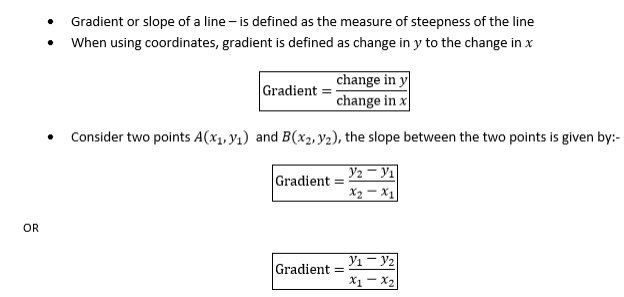Example 1
Find the gradient of the lines joining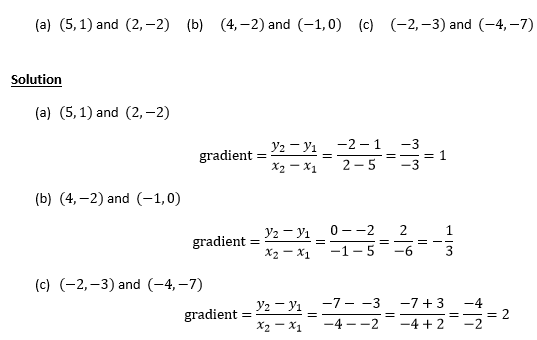Example 2
(a) The line joining (2, -3) and (k, 5) has a gradient -2. Find k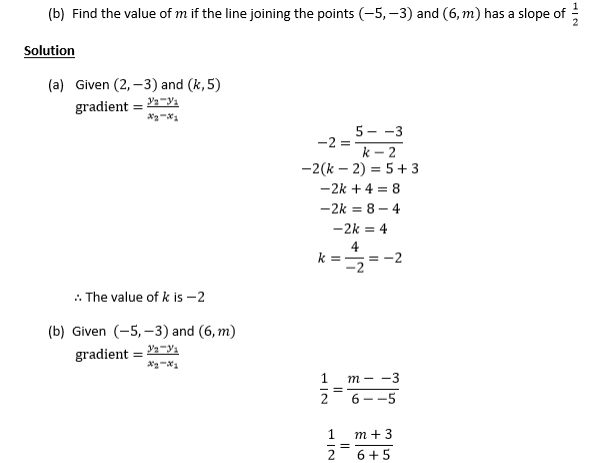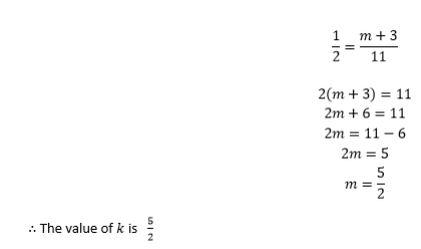Exercise 1
1. Find the gradientof the line which passes through the following points ;
1. (3,6) and (-2,8)
2. (0,6) and (99,-12)
3. (4,5)and (5,4)
2. A line passes through (3, a) and (4, -2), what is the value of a if the slope of the line is 4?
3. The gradient of the linewhich goes through (4,3) and (-5,k) is 2. Find the value of k.
FINDING THE EQUATION OF A STRAIGHT LINE
The equation of a straight line can be determined if one of the following is given:-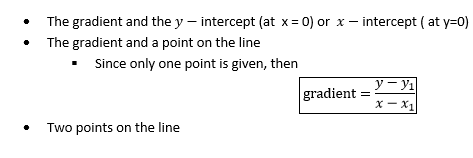Example 3
Find the equation of the line with the following
2. Gradient and passing through the point
3. Passing through the points and
Solution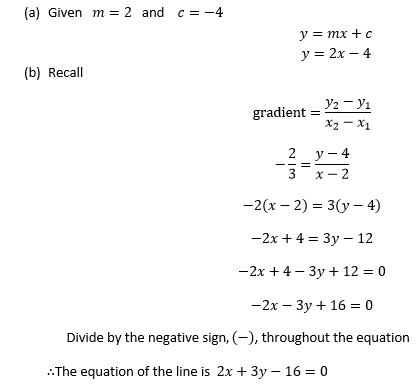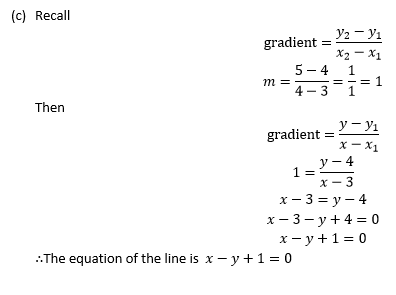EQUATION OF A STRAIGHT LINE IN DIFFERENT FORMS
The equation of a line can be expressed in two forms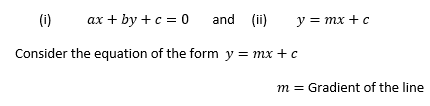Example 4
Find the gradient of the following lines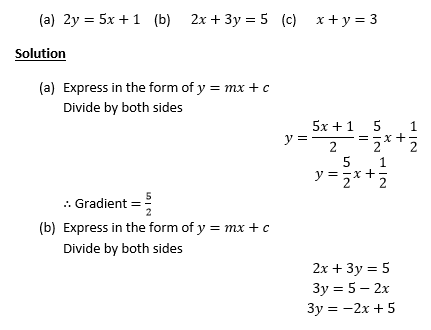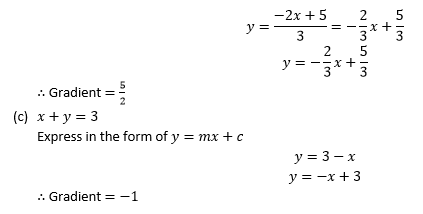INTERCEPTS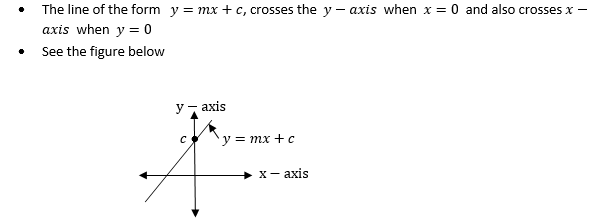Therefore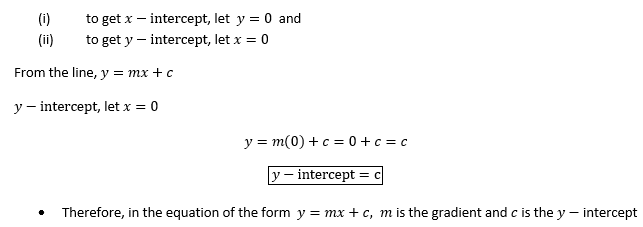Example 5
Find the y-intercept of the following lines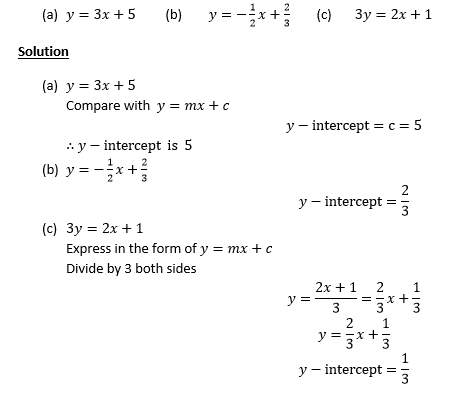Example 6
Find the x and y-intercept of the following lines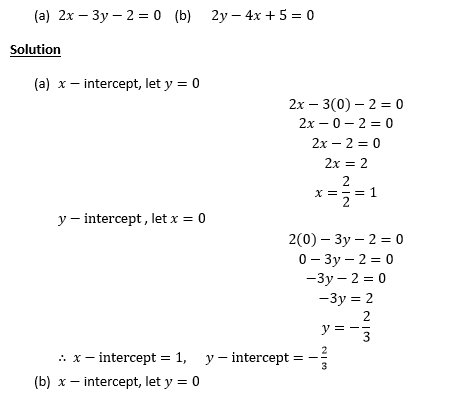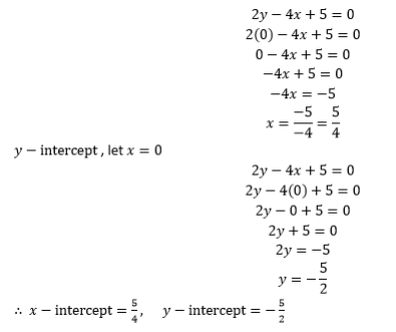Exercise 2
Attempt the following Questions.
1. Find the y-intercept of the line 3x+2y = 18 .
2. What is the x-intercept of the line passing through (3,3) and (-4,9)?
3. Calculate the slope of the line given by the equation x-3y= 9
4. Find the equation of the straight line with a slope -4 and passing through the point (0,0).
5. Find the equation of the straight line with y-intercept 5 and passing through the point (-4,8).
GRAPHS OF STRAIGHT LINES
The graph of straight line can be drawn by using the following methods;
1. By using intercepts
2. By using the table of values
Example 7
Sketch the graph of Y = 2X – 1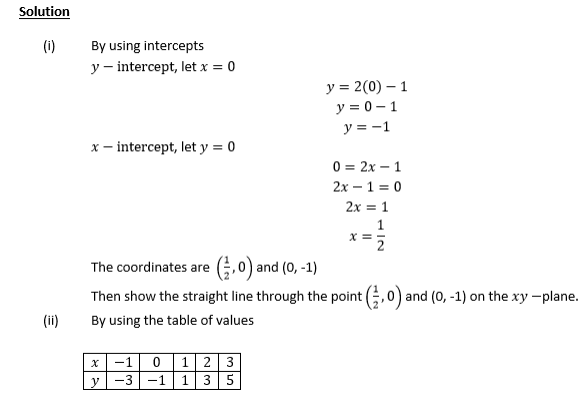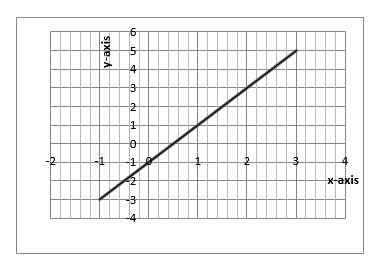SOLVING SIMULTANEOUS EQUATION BY GRAPHICAL METHOD
• Use the intercepts to plot the straight lines of the simultaneous equations
• The point where the two lines cross each other is the solution to the simultaneous equations
Example 8
Solve the following simultaneous equations by graphical methodExercise 3
1. Draw the line 4x-2y=7 and 3x+y=7 on the same axis and hence determine their intersection point
2. Find the solutionfor each pair the following simultaneous equations by graphical method;
1. y-x = 3 and 2x+y = 9
2. 3x- 4y=-1 and x+y = 2
3. x = 8 and 2x-3y = 10